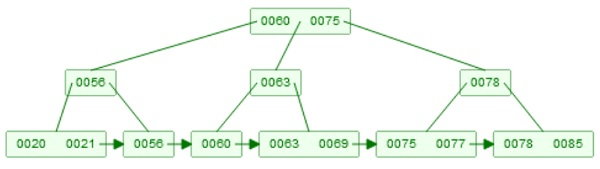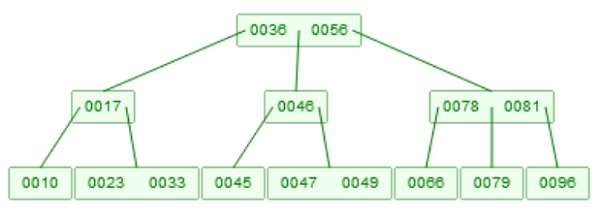# B+ tree Insertion in Data Structure

Here we will see, how to perform the insertion into a B+ Tree. Suppose we have a B+ Tree like below −

Example of B+ TreeTo insert an element, the idea is very similar to the B-Tree, if one element is inserted, that will be stored at the leaf node. If that is present in some internal node, then it will also be there, at the leaf as right child of itself.

Suppose we want to insert 65 into the tree. So that is greater than 60, and less than 75. Then it will be inserted into the middle sub-tree. Now, 65, will be inserted into node after 63, then that node will be divided into two parts, 65 will go up, and 65 will also be there at the right node of it.

B+ Tree after inserting 65.## Algorithm

BPlusTreeInsert(root, key)

Input − The root of the tree, and key to insert

We will assume, that the key is not present into the list
Start from root node, perform exact match for key as ‘key’ till a leaf node. Let the search path
be x1, x2, … , xh. The x1 is first node so root, then xh is leaf node. Each node xi is parent of xi+1
Insert the new object where key is ‘key’, and value is v into xh.
i := h
while xi overflows, do
divide xi into two nodes, by moving the larger half of the keys into a new node p.
if xi is leaf node, link p into the linked list among leaf nodes.
identify a key k, to be inserted into the parent level along with child pointer pointing p.
The choice of k depends on the type of the node xi. If xi is leaf node, we will perform
copy up. So smallest key in p, is copied as k to the parent level. On the other hand, if xi is
non-leaf node, then we will perform push up. So smallest key in p, will be copied into k,
in the parent node.
if i = 0, then
create a new index node as the new root. In the new root store node with key k,
and two child xi and p.
return
else
insert a key k and a child pointer pointing to p, into node xi-1.
i := i – 1
end if
done

Updated on: 11-Aug-2020

254 Views